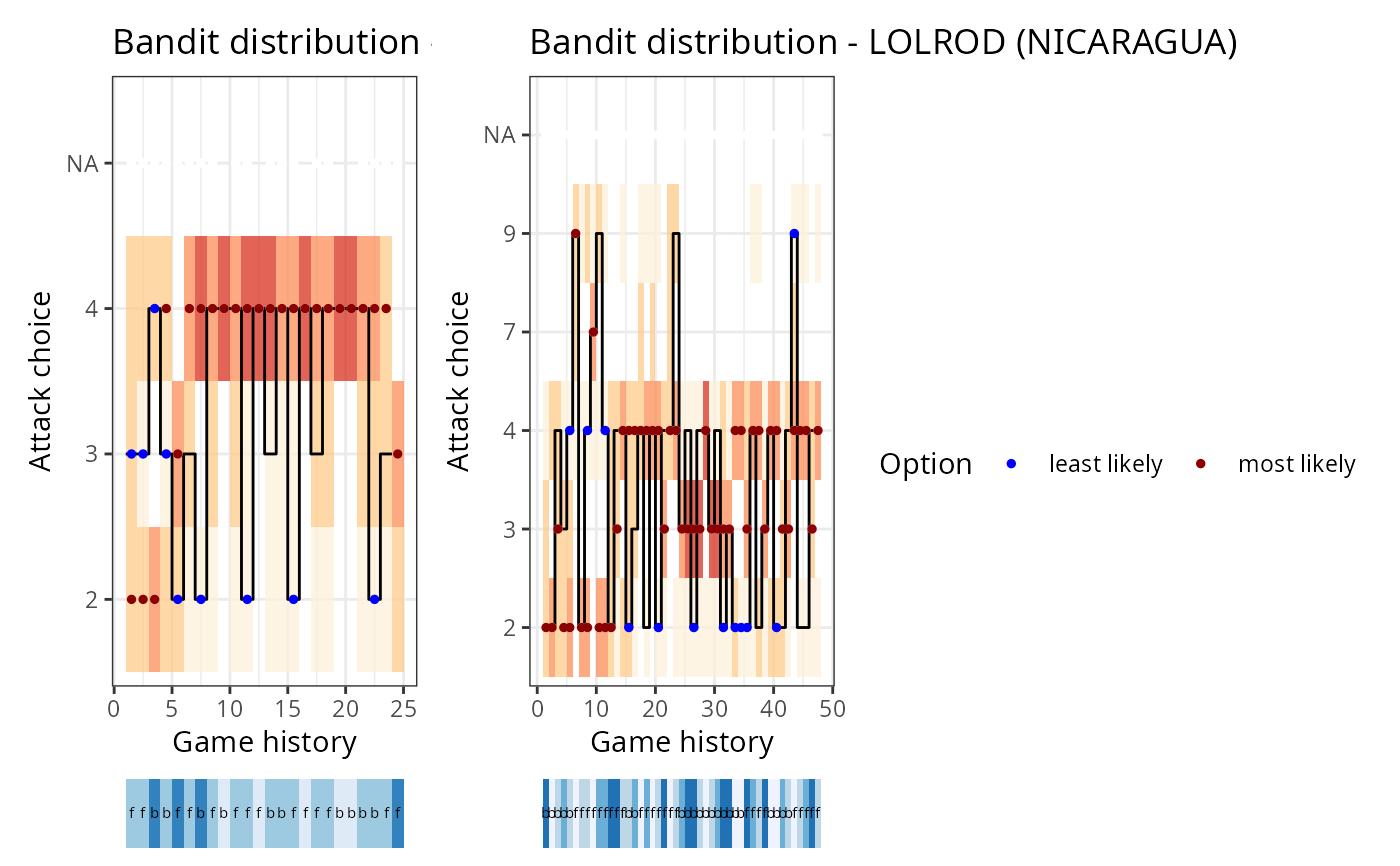Plot a simulated setter distribution sequence

## Usage

ov_plot_sequence_distribution(
ssd,
label_setters_by = "id",
font_size = 11,
title_wrap = NA,
split_set = FALSE,
output = "plot"
)

## Arguments

ssd

simulated setter distribution output as returned by ov_simulate_setter_distribution()

label_setters_by

string: either "id" or "name"

font_size

numeric: font size

title_wrap

numeric: if non-NA, use strwrap() to break the title into lines of this width

split_set

boolean: if TRUE, separate the distribution sequence by set

output

string: either "plot" or "list"

## Examples

dvw <- ovdata_example("190301_kats_beds")
ssd <- ov_simulate_setter_distribution(dvw = dvw, play_phase = c("Reception"),
n_sim = 100, attack_by = "zone",
setter_position_by = "front_back")
ov_plot_sequence_distribution(ssd)
#> Warning: Removed 89 rows containing missing values (geom_point()).
#> Warning: Removed 110 rows containing missing values (geom_point()).